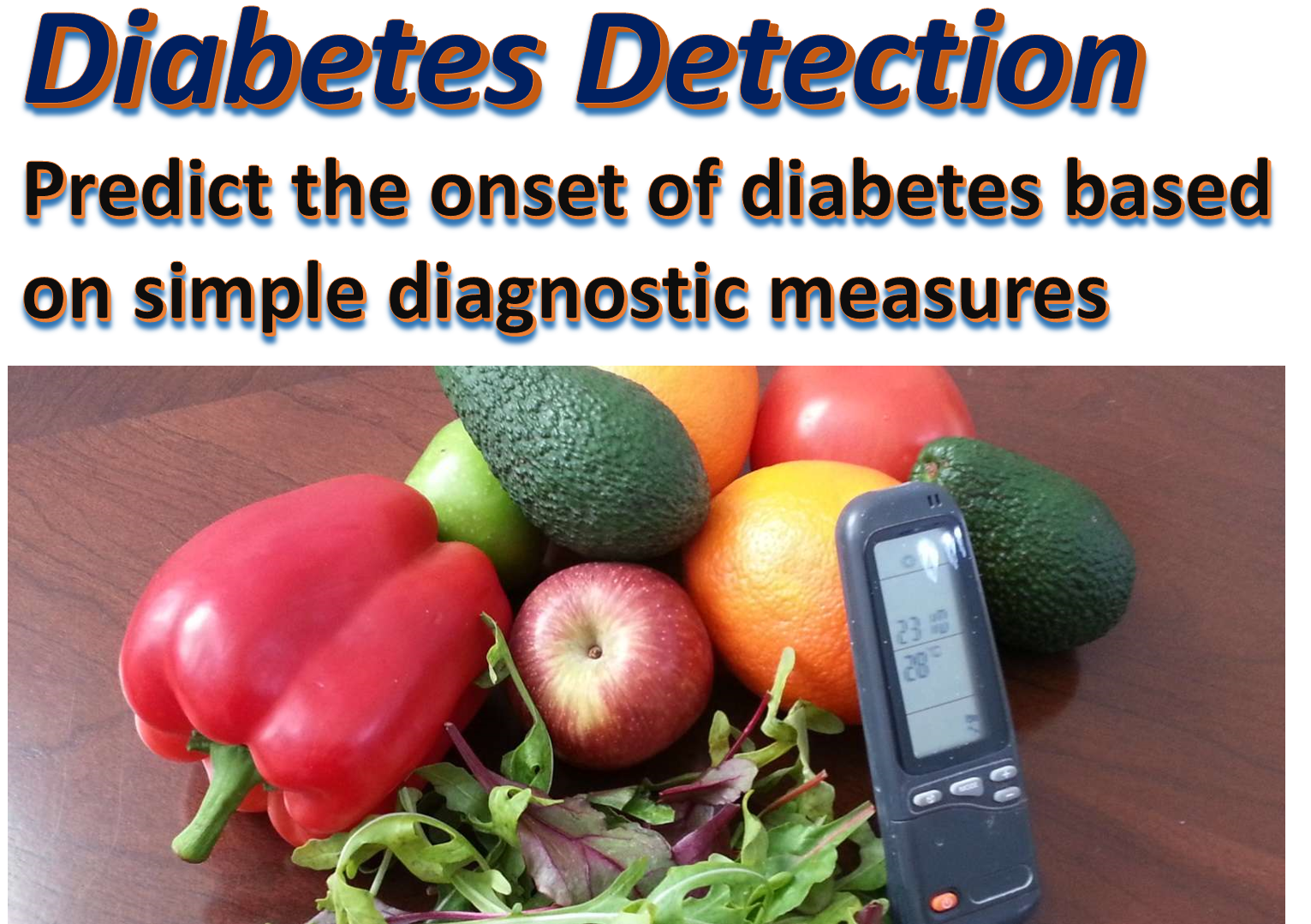This database is used as a cannonical example for several topics in data science and machine learning. The Pima indian diabetes dataset is originally from the National Institute of Diabetes and Digestive and Kidney Diseases. The objective is to predict if a patient has diabetes based on diagnostic measurements of eight simple features. We will also use this dataset for learning the basics of the Pandas library (which is essential for data science and machine learning).

Let's first start with listing the required Python modules for this project. We use the Keras deep learning library which makes things simple and practical. In a subsequent study unit we will also show how this can be done with Tensorflow.

Also note that we are using a designated module kerutils which contains some useful Keras and Numpy utilities for all our course units. It can be downloaded from: https://github.com/samyzaf/kerutils

In :
from keras.models import Sequential, load_model
from keras.layers import Dense, Dropout, Activation, Flatten
from keras.layers.advanced_activations import PReLU, SReLU, ELU, LeakyReLU, ThresholdedReLU, ParametricSoftplus
from keras.layers.noise import GaussianNoise
from keras.utils.visualize_util import plot
import matplotlib.pyplot as plt
import matplotlib.cm
import pandas as pd
from pandas.tools.plotting import scatter_matrix
from kerutils import *

from matplotlib import rcParams
rcParams['axes.grid'] = True
rcParams['figure.figsize'] = 10,7
%matplotlib inline

# fixed random seed for reproducibility
np.random.seed(0)
from kerutils import *

In :
# These are css/html styles for good looking ipython notebooks
from IPython.core.display import HTML
HTML('<style>{}</style>'.format(css))

Out:
In :
features = ['preg', 'plas', 'pres', 'skin', 'test', 'mass', 'pedi', 'age', 'class']

In :
# Lets view the first 10 rows of the data set
# See below what these names mean


Out:
preg plas pres skin test mass pedi age class
0 6 148 72 35 0 33.6 0.627 50 1
1 1 85 66 29 0 26.6 0.351 31 0
2 8 183 64 0 0 23.3 0.672 32 1
3 1 89 66 23 94 28.1 0.167 21 0
4 0 137 40 35 168 43.1 2.288 33 1
5 5 116 74 0 0 25.6 0.201 30 0
6 3 78 50 32 88 31.0 0.248 26 1
7 10 115 0 0 0 35.3 0.134 29 0
8 2 197 70 45 543 30.5 0.158 53 1
9 8 125 96 0 0 0.0 0.232 54 1

### Medical Features short names¶

1. preg = Number of times pregnant
2. plas = Plasma glucose concentration a 2 hours in an oral glucose tolerance test
3. pres = Diastolic blood pressure (mm Hg)
4. skin = Triceps skin fold thickness (mm)
5. test = 2-Hour serum insulin (mu U/ml)
6. mass = Body mass index (weight in kg/(height in m)^2)
7. pedi = Diabetes pedigree function
8. age = Age (years)
9. class = Class variable (1:tested positive for diabetes, 0: tested negative for diabetes)

In :
# How many rows do we have?

data.index

Out:
RangeIndex(start=0, stop=768, step=1)
In :
# Try2: How many rows do we have?

len(data.index)

Out:
768
In :
# How many columns do we have?

len(data.columns)

Out:
9
In :
# How many records do we have in our data set?

data.size    # 9 * 768

Out:
6912
In :
# You can get everything in one line !

data.shape

Out:
(768, 9)
In :
# View the last 10 records of our data set

data.tail(10)

Out:
preg plas pres skin test mass pedi age class
758 1 106 76 0 0 37.5 0.197 26 0
759 6 190 92 0 0 35.5 0.278 66 1
760 2 88 58 26 16 28.4 0.766 22 0
761 9 170 74 31 0 44.0 0.403 43 1
762 9 89 62 0 0 22.5 0.142 33 0
763 10 101 76 48 180 32.9 0.171 63 0
764 2 122 70 27 0 36.8 0.340 27 0
765 5 121 72 23 112 26.2 0.245 30 0
766 1 126 60 0 0 30.1 0.349 47 1
767 1 93 70 31 0 30.4 0.315 23 0
In :
# The plot() method plots all features
# This is TMI (too much information)

data.plot()

Out:
<matplotlib.axes._subplots.AxesSubplot at 0x18e66a67ef0>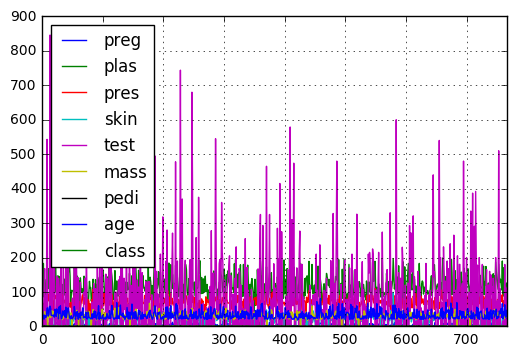In :
# Better try one at a time
# Here is the plasma concentration level per record distribution
# (less is more!)

data.plot(y='plas')

Out:
<matplotlib.axes._subplots.AxesSubplot at 0x18e67ec9b70>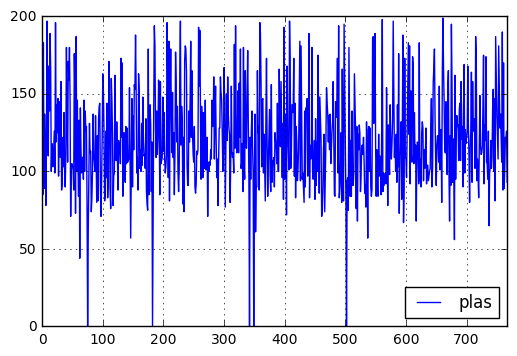In :
# The graph above does not look very useful.
# Let's sort the plas values
plt.plot(sorted(data.plas.as_matrix()))

Out:
[<matplotlib.lines.Line2D at 0x18e67fbdb70>]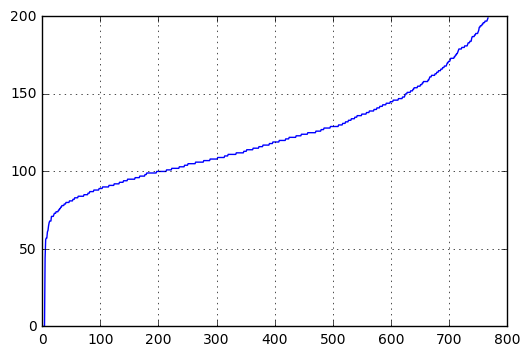In :
# This is the age distribution (sorted!)

plt.plot(sorted(data.age.as_matrix()))

Out:
[<matplotlib.lines.Line2D at 0x18e680259b0>]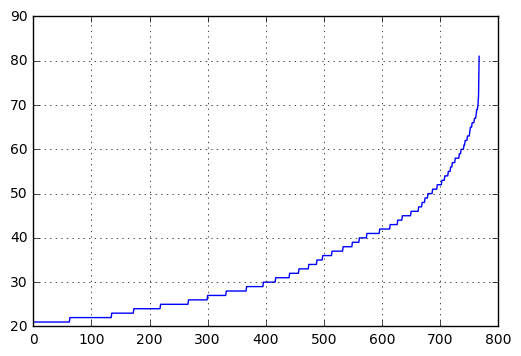In :
# Age range

print("Min age = %d, Max age = %d" % (data.age.min(), data.age.max()))

Min age = 21, Max age = 81

In :
# Age average

data.age.mean()

Out:
33.240885416666664
In :
# Age median

data.age.median()

Out:
29.0
In :
top = plt.subplot2grid((4,4), (0, 0), rowspan=2, colspan=4)
top.scatter(data['plas'], data['age'])
plt.title('Age against Plasma')
bottom = plt.subplot2grid((4,4), (2,0), rowspan=2, colspan=4)
bottom.bar(data['preg'], data['plas'])
plt.title('Plasma against Pregnancies')
plt.tight_layout()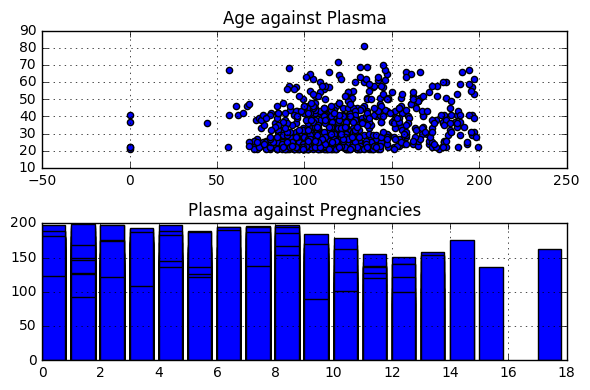## Distribution by class=0 and class=1 (negative/positive diabetes test)¶

In :
# Items with class == 0 (negative diabetes check)

data0 = data[data['class'] == 0]
len(data0.index)

Out:
500
In :
# Items with class == 1 (persons with a positive diabtetes check)

data1 = data[data['class'] == 1]
len(data1.index)

Out:
268
In :
# The Pandas groupby method computes the distribution of one feature
# with respect to the others
# We see 8 histograms distrubuted against a negative diabetes chck
# and other 8 histograms with distribution against a positive diabetes check

data.groupby('class').hist(figsize=(8,8), xlabelsize=7, ylabelsize=7)

Out:
class
0    [[Axes(0.125,0.684722;0.215278x0.215278), Axes...
1    [[Axes(0.125,0.684722;0.215278x0.215278), Axes...
dtype: object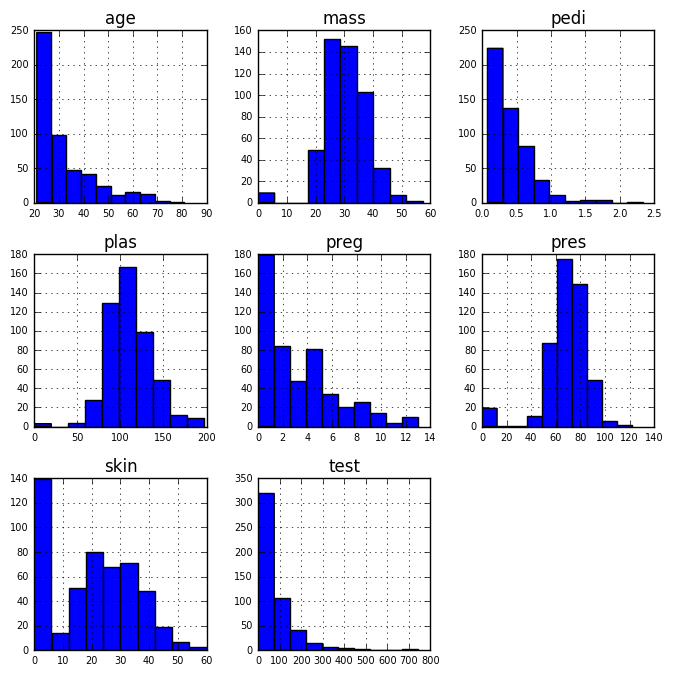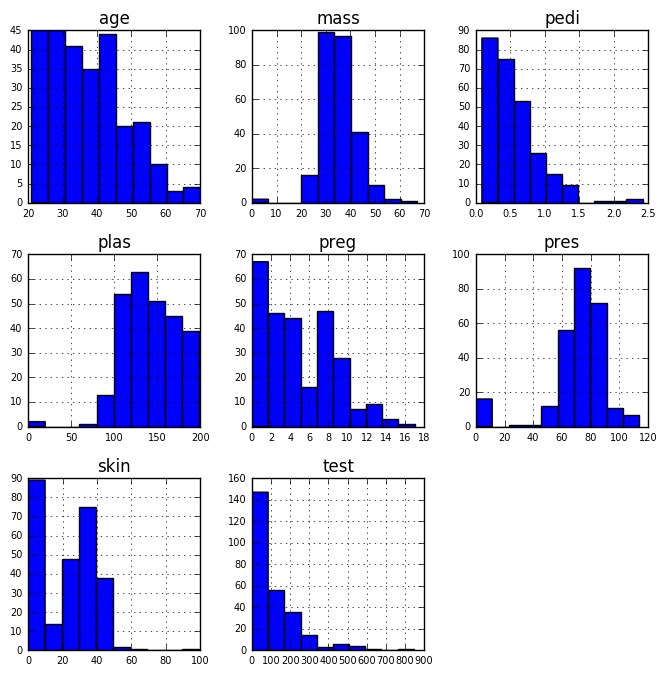In :
sm = scatter_matrix(data, alpha=0.2, figsize=(7.5, 7.5), diagonal='kde')

[plt.setp(item.yaxis.get_majorticklabels(), 'size', 6) for item in sm.ravel()]
[plt.setp(item.xaxis.get_majorticklabels(), 'size', 6) for item in sm.ravel()]